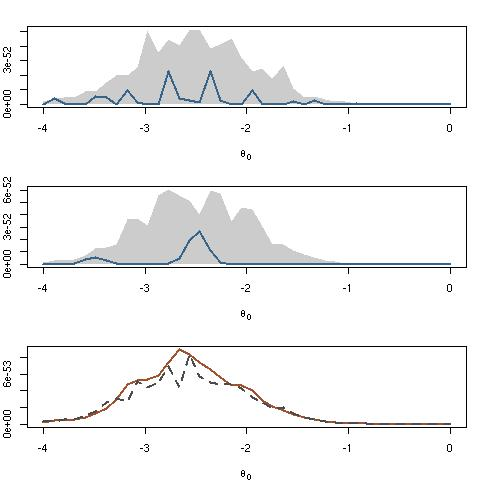Want to share your content on R-bloggers? click here if you have a blog, or here if you don't.The function pimax from our package mcsm is used in to reproduce Figure 5.11 of our book Introducing Monte Carlo Methods with R. (The name comes from using the Pima Indian R benchmark as the reference dataset.) I got this email from Josué

I ran the ‘pimax’ example from the mcsm manual, and it gave me the following message:

```> pimax(Nsim = 10^3)
Error in raaj[t, ] = apply(as.matrix(aas), 1, margap) :
number of items to replace is not a multiple of replacement length
> pimax()
Error in raaj[t, ] = apply(as.matrix(aas), 1, margap) :
number of items to replace is not a multiple of replacement length```

but when running pimax(10^2) on my machine I did get the following picture and no error message. So I wonder if this is a matter of version of R or something else…Filed under: Books, R, Statistics Tagged: Introducing Monte Carlo Methods with R, mcsm, Pima Indians, pimax, R